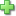# Math-U-See Algebra 2 - Student Pack

Publisher: Math-U-See
2nd Edition, ©2009, Publisher Catalog #1131
Price: \$48.00

See series description for full review.

# Algebra 2

### Factoring Polynomials, Quadratic Formula, Graphing Conic Sections, and Other Algebra 2 Topics

Algebra 2 is a more rigorous algebra course that builds on the previous two courses. Besides review and expansion of topics taught in Algebra 1, the course covers advanced factoring, imaginary and complex numbers, conjugate numbers, the binomial theorem, the quadratic formula, motion problems, and other kinds of application problems. Graphing includes extensive practice with conic sections and solving systems of equations visually and algebraically. Vectors are also introduced.

## Student Pack

For customers who already have the needed instructor materials.

The Student Pack includes:

• Student Workbook
• Tests booklet

Each student will need their own Student Workbook and Tests booklet.

Scope and Sequence:

• Lesson 01: Exponents
• Lesson 02: Rational Expressions
• Lesson 03: Scientific Notation; Combining Like Terms
• Lesson 04: Radicals: Basic Operations and Simplifying
• Lesson 05: Factoring Polynomials; Rational Expressions
• Lesson 06: Fractional Exponents
• Lesson 07: Imaginary and Complex Numbers
• Lesson 08: Conjugate Numbers
• Lesson 09: Squares, Cubes, and Pascal’s Triangle
• Lesson 10: Binomial Theorem
• Lesson 11: Completing the Square
• Lesson 13: Discriminants
• Lesson 14: Applications Using Percent
• Lesson 15: Isolating a Variable
• Lesson 16: Ratios
• Lesson 17: Unit Multipliers and Metric Conversions
• Lesson 18: Distance = Rate x Time
• Lesson 19: Motion Problems
• Lesson 20: Graphing Lines: Slope-Intercept Formula
• Lesson 21: Graphing Parallel and Perpendicular Lines; Graphing Inequalities
• Lesson 22: Distance Formula and Midpoint Formula
• Lesson 23: Conic Sections: Circle and Ellipse
• Lesson 24: Conic Sections: Parabola
• Lesson 25: Parabola: Maxima and Minima
• Lesson 26: Conic Sections: Hyperbola
• Lesson 27: Solving Systems of Equations: Lines and Conic Sections
• Lesson 28: Coin Problems, Consecutive Integers, and Chemical Mixtures
• Lesson 29: Age and Boat-in-the-Current Problems
• Lesson 30: Solving Equations with Three Variables
• Lesson 31: Vectors

## Sample Lesson

Did you find this review helpful?
Series Description
Related CategoriesClick here to write a review